# Meso Level Analysis: Focusing on Countries¶

Let's start by importing all of the necessary libraries to conduct the analysis.

In :
from py2neo import Graph
import numpy as np
from pandas import DataFrame
import itertools
import matplotlib.pyplot as plt
import seaborn as sns
import json
import math
import pandas as pd
import plotly
import plotly.graph_objs as go
import qgrid
from scipy import stats, spatial
from sklearn.cluster.bicluster import SpectralBiclustering
import operator
from IPython.display import display, HTML
from matplotlib.colors import ListedColormap
from wordcloud import WordCloud

local_connection_url = "http://localhost:7474/db/data"
connection_to_graph = Graph(local_connection_url)



## 1. Characterization of countries ¶

In this part of the analysis, severall co-occurence matrixes will be produced. Ideally, one for every country in the database.

### 1.1. Co-ocurence matrixes for countries ¶

We start by getting a list of all of the countries in the neo4j database.

In :
country_query = """ MATCH (n:Country)
WITH n.name AS Country
RETURN Country;
"""

country_names = list(set(DataFrame(connection_to_graph.data(country_query)).as_matrix()[:, 0]))
country_names.sort()

print 'The country list has {} countries.'.format(len(country_names))

The country list has 145 countries.

In :
f_terms = list(set(DataFrame(connection_to_graph.data('MATCH (a:Asset)-[:CONTAINS]->(fs:Feedstock) RETURN  fs.term, count(a)')).as_matrix()[:, 1]))
o_terms = list(set(DataFrame(connection_to_graph.data('MATCH (a:Asset)-[:CONTAINS]->(fs:Output) RETURN  fs.term, count(a)')).as_matrix()[:, 1]))
pt_terms = list(set(DataFrame(connection_to_graph.data('MATCH (a:Asset)-[:CONTAINS]->(fs:ProcessingTech) RETURN  fs.term, count(a)')).as_matrix()[:, 1]))
bbo = list(set(f_terms + pt_terms + o_terms))
print len(bbo)
matrix_axis_names = bbo

352


After doing this, we prepare a function that given a certain country, will retrieve the co-occurence matrix. This process is similar to the process in "1.3. The first part of the matrix: No intersections" but applied to a particular country. By aggregating the process done before in a single function.

In :
def get_country_matrix(country, normalization=True):

# define queries
country_no_interestions = """   MATCH (a:Asset)-[:CONTAINS]->(fs:Feedstock)
MATCH (a:Asset)-[:CONTAINS]->(out:Output)
MATCH (a:Asset)-[:CONTAINS]->(pt:ProcessingTech)
WHERE a.country = "{}"
RETURN fs.term, pt.term, out.term, count(a)
""".format(country)

process_variables = ['Feedstock', 'Output', 'ProcessingTech']

country_intersections = """     MATCH (a:Asset)-[:CONTAINS]->(fs:{})
MATCH (a:Asset)-[:CONTAINS]->(t:{})
WHERE fs<>t AND a.country = "{}"
RETURN fs.term, t.term, count(a)
"""
if country == 'root':
total_documents = 1
else:

q_documents = """   MATCH (n:Asset)-[:LOCATED_IN]->(c:Country)
WHERE c.name = "{}"
RETURN c, count(n)""".format(country)

total_documents = DataFrame(connection_to_graph.data(q_documents)).as_matrix()

# get data
data_no_intersections = DataFrame(connection_to_graph.data(country_no_interestions)).as_matrix()

# create matrix
country_matrix = np.zeros([len(matrix_axis_names), len(matrix_axis_names)])

# for no intersections data
for row in data_no_intersections:
# the last column is the frequency (count)
frequency = row
indexes = [matrix_axis_names.index(element) for element in row[1::]]
# add frequency value to matrix position
for pair in itertools.combinations(indexes, 2):
country_matrix[pair, pair] += frequency
country_matrix[pair, pair] += frequency

# for intersecting data
for category in process_variables:
process_data = DataFrame(connection_to_graph.data(country_intersections.format(category, category, country))).as_matrix()
for row in process_data:
frequency = row
indexes = [matrix_axis_names.index(element) for element in row[1::]]
# add frequency value to matrix position
for pair in itertools.combinations(indexes, 2):
country_matrix[pair, pair] += frequency / 2 # Divided by two because query not optimized
country_matrix[pair, pair] += frequency / 2 # Divided by two because query not optimized

# normalize
#normalized_country_matrix = (country_matrix - np.mean(country_matrix)) / np.std(country_matrix)

normalized_country_matrix = country_matrix / total_documents

# dynamic return
if normalization == True:
return normalized_country_matrix
else:
return country_matrix



Let's create a function that returns basic stats given a matrix. With this function, we can gain insight into the previous function.

In :
def basic_stats(a_matrix):
print 'Rows:', a_matrix.shape
print 'Columns:', a_matrix.shape
print 'Mean: ', np.mean(a_matrix)
print 'Standart Deviation', np.std(a_matrix)
print 'Max: ', np.amax(a_matrix)
print 'Min: ', np.amin(a_matrix)
print 'Symmetry: ', check_symmetric(a_matrix, 1e-8)
print ''

def check_symmetric(a, tol):
return np.allclose(a, a.T, atol=tol)


Let's test a couple of countries. By getting their co-occurence matrix and printing its properties.

In :
print 'Denmark co-occurence matrix stats:'
basic_stats(get_country_matrix('Denmark', normalization=True))
print 'Sweden co-occurence matrix stats:'
basic_stats(get_country_matrix('Sweden', normalization=True))

Denmark co-occurence matrix stats:
Rows: 352
Columns: 352
Mean:  3.655987320760048e-05
Standart Deviation 0.0008689242584471302
Max:  0.08831908831908832
Min:  0.0
Symmetry:  True

Sweden co-occurence matrix stats:
Rows: 352
Columns: 352
Mean:  5.3249688971829727e-05
Standart Deviation 0.0008832382504540617
Max:  0.1010752688172043
Min:  0.0
Symmetry:  True


In :
def borders(width, color):
plt.axhline(y=0, color='k',linewidth=width)
plt.axhline(y=get_country_matrix('Denmark', normalization=True).shape, color=color,linewidth=width)
plt.axvline(x=0, color='k',linewidth=width)
plt.axvline(x=get_country_matrix('Denmark', normalization=True).shape, color=color,linewidth=width)

In :
# create subplots
plt.subplots(2,1,figsize=(17,17))

bwhite = 'binary'
vmax = 0.01
vmin=0.00

plt.subplot(121)
sns.heatmap(get_country_matrix('Denmark', normalization=True),cbar=True, cbar_kws={"shrink": .2}, cmap=bwhite, square=True, xticklabels=False, yticklabels=False, vmin=vmin, vmax=vmax)
borders(1.5, 'k')
plt.title('Normalized Capability Matrix: Denmark')

plt.subplot(122)
sns.heatmap(get_country_matrix('Sweden', normalization=True), cbar=True, cbar_kws={"shrink": .2}, cmap=bwhite, square=True, xticklabels=False, yticklabels=False, vmin=vmin, vmax=vmax)
borders(1.5, 'k')
plt.title('Normalized Capability Matrix: Sweden')
plt.show()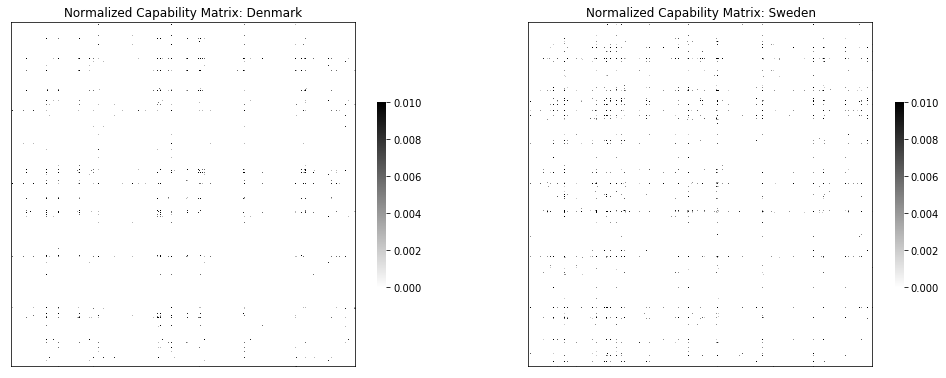### 1.2. Country capability lists ¶

One of the goals of the analysis is to understand how each country relates to another. To do this, we will need to transform the matrix of a given country into an array.

After doing this we will be able to compare the array of each one of the countries, by computing their difference or correlation for example.

Let's start by creating a function that given a symetric matrix, as the ones shown above, returns a list. This list will have an entry by position in the matrix. But since the matrixes are symmetrical, the list will only receive half of the matrix.

This means that for a matrix of dimensions 342x342 the list will have a total of 58 482 entries.

In :
def get_list_from(matrix):
total_rows = matrix.shape
only_valuable = []
extension = 1
for row_number in range(total_rows):
only_valuable.append(matrix[row_number, extension:total_rows].tolist())
extension += 1
return [element for column in only_valuable for element in column ]


Let's visualize the lists produced by this workflow for two different countries.

We first select two countries.

In :
spectrum_countries = ['''People's Republic of China''', 'United States of America']


We then create a matrix where each row is the vector that describes the country's capabilities.

In :
# apply functions to both countries
country_1_list = get_list_from(get_country_matrix(spectrum_countries, normalization=True))
country_2_list = get_list_from(get_country_matrix(spectrum_countries, normalization=True))

# create a matrix where each row is a list of a country
corelation = np.vstack((country_1_list, country_2_list))

# plot the matrix
good_cols = [i for i in range(corelation.shape) if np.sum(corelation[:, i]) != 0]

good_corelation = corelation[:, good_cols]
print good_corelation.shape

plt.subplots(1,1,figsize=(20, 5))
plt.subplot(111)
sns.heatmap(good_corelation,cmap=ListedColormap(['white', 'black']), center=0.000001, cbar=None, square=False, yticklabels=[spectrum_countries, spectrum_countries], xticklabels=False)
plt.yticks(rotation=0)
plt.title('Country Capability Spectrum', size=15)
plt.show()

(2, 4920)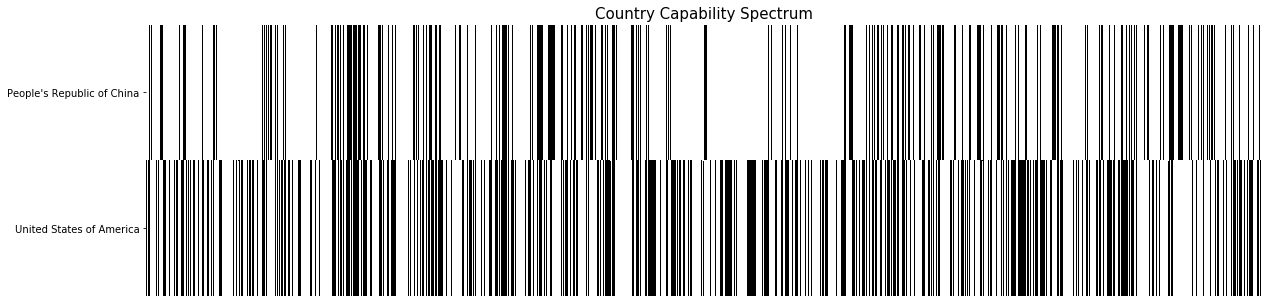We can see that the spectrum differs significantly in some areas. Please note that all of the measure were normalized prior to the plotting.

## 2. Country Correlation Matrix and Profiles ¶

### 2.1. Regular country correlation matrix ¶

In this part of the analysis we will start correlating countries in relation to their capabilities.

The correlation matrix follows the following principle:

• Entry (i, j) is the correlation index between country i and country j
• The correlation index between country i and j is the absolute average difference between the capability list of country i and j.

Now, taking the list of countries previously established, we can iterate through it and fill the matrix.

To improve eficiency, we first create a dictionnary where each key is a country, and each value, the capability list.

In the case that the databse contains no assets about a certain country, that country will be discarted.

In :
# create dictionnary
country_capability_dict = {}
counter = 0

# iterate through countries
for country in country_names:
counter += 1
country_matrix = get_country_matrix(country, normalization=True)
list_ = get_list_from(country_matrix)
sum_of_list = sum(list_)

# discart if no information
if np.all(np.isnan(country_matrix)) or sum_of_list == 0.0:
continue
else:
country_capability_dict[country] = get_list_from(country_matrix)

np.save('Data/country_capability_dict.npy', country_capability_dict)

In :
country_names = country_capability_dict.keys()
country_names.sort()
number_of_countries = len(country_names)

print 'Here is the list of {} countries:'.format(number_of_countries)
print country_names

print '\nHere is the list of {} countries with unsuficient information:'.format(len(bad_country_list))

Here is the list of 76 countries:
[u'Argentina', u'Asia', u'Australia', u'Austria', u'Bangladesh', u'Belgium', u'Bosnia and Herzegovina', u'Brazil', u'Canada', u'Chile', u'Colombia', u'Costa Rica', u'Cyprus', u'Czech Republic', u'Denmark', u'Dominion of Pakistan', u'Egypt', u'El Salvador', u'Estonia', u'European Patent Office', u'Finland', u'France', u'Germany', u'Greece', u'Guatemala', u'Hong Kong', u'Hungary', u'India', u'Indonesia', u'Iran', u'Iraq', u'Ireland', u'Israel', u'Italy', u'Japan', u'Korea', u'Kuwait', u'Latvia', u'Lebanon', u'Lithuania', u'Malaysia', u'Mexico', u'Netherlands', u'New Zealand', u'Nigeria', u'Norway', u'Null', u"People's Republic of China", u'Philippines', u'Poland', u'Portugal', u'Romania', u'Russia', u'Saudi Arabia', u'Scotland', u'Serbia', u'Singapore', u'Slovakia', u'Slovenia', u'South Africa', u'South Korea', u'Spain', u'Sweden', u'Switzerland', u'Taiwan', u'Thailand', u'Tunisia', u'Turkey', u'Uganda', u'Ukraine', u'United Arab Emirates', u'United Kingdom', u'United States of America', u'Vietnam', u'WO', u'Zimbabwe']

Here is the list of 69 countries with unsuficient information:
[u'Albania', u'Algeria', u'Armenia', u'Azerbaijan', u'Belarus', u'Benin', u'Bolivia', u'Botswana', u'Brunei', u'Bulgaria', u'Burkina Faso', u'Burundi', u'Cambodia', u'Cameroon', u'Cape Verde', u'Central African Republic', u'Croatia', u'Cuba', u'Dominican Republic', u'Ecuador', u'Ethiopia', u'Falkland Islands', u'Faroe Islands', u'Fiji', u'Georgia', u'Ghana', u'Guinea', u'Honduras', u'Iceland', u'Jamaica', u'Jordan', u'Kazakhstan', u'Kenya', u'Libya', u'Liechtenstein', u'Luxembourg', u'Macedonia', u'Madagascar', u'Malawi', u'Mali', u'Malta', u'Mauritius', u'Moldova', u'Montenegro', u'Morocco', u'Mozambique', u'Namibia', u'Nepal', u'Nicaragua', u'North Korea', u'Oman', u'Pakistan', u'Panama', u'Paraguay', u'Peru', u'Qatar', u'Republic of Macedonia', u'Saint Vincent and the Grenadines', u'Senegal', u'Sri Lanka', u'Sudan', u'Swaziland', u'Tanzania', u'Togo', u'Trinidad and Tobago', u'Uruguay', u'Venezuela', u'Yemen', u'Zambia']


Let's create a function that given two countries and a method, returns a suitable correlation coeficient.

Here, the code will give three possible outputs:

• abs_avg_dif: the absolute average difference between two vectors.
• Pearson: The pearson correlation coeficient between two vectors.
• P-value: The p-value according to scipy.
In :
def calculate_country_correlation(country1_list, country2_list, stat):
avg_dif = np.mean(country1_list - country2_list)
abs_avg_dif = abs(avg_dif)

if stat.lower() == 'absolute average difference': # return absolute average difference
return abs_avg_dif
if stat == 'Pearson':                             # return Pearson coef
return stats.pearsonr(country1_list, country2_list)
if stat == 'P-value':                             # return P-value
return stats.pearsonr(country1_list, country2_list)


The matrix is built, with the following steps:

1. For each country, get its asset co-occurence matrix.
2. Normalize the matrix by subtracting the mean and dividing by the standard deviation.
3. Transform the matrix into a list by taking its upper triangle.
4. For each entry (i,j) of the matrix, calculate the correlation of the list of country i and the list of country j.
In :
country_correlation = np.zeros([number_of_countries, number_of_countries])

for row in range(number_of_countries):

country_1 = country_names[row]
country_1_list = np.asarray(country_capability_dict[country_1])
for column in range(number_of_countries):
country_2 = country_names[column]
country_2_list = np.asarray(country_capability_dict[country_2])

country_correlation[row, column] = calculate_country_correlation(country_1_list, country_2_list, 'Pearson')

np.save('Data/country_correlation.npy', country_correlation)
np.save('Data/country_names.npy', country_names)

In :
print 'Minimum correlation value is {} for countries {} and {}.'.format(country_correlation[np.unravel_index(country_correlation.argmin(), country_correlation.shape),np.unravel_index(country_correlation.argmin(), country_correlation.shape)],country_names[np.unravel_index(country_correlation.argmin(), country_correlation.shape)], country_names[np.unravel_index(country_correlation.argmin(), country_correlation.shape)])

Minimum correlation value is -0.00100362058212 for countries Israel and Netherlands.


After building the matrix, we create the first heatmap of that matrix using the sns.heatmap function.

In :
plt.subplots(1,1,figsize=(15, 15))
plt.subplot(111)
sns.heatmap(country_correlation, cbar=True,cbar_kws={"shrink": .5} ,square=True, yticklabels=country_names, xticklabels=country_names)
plt.title('Country Correlation Matrix: Unordered', size=13)
plt.show()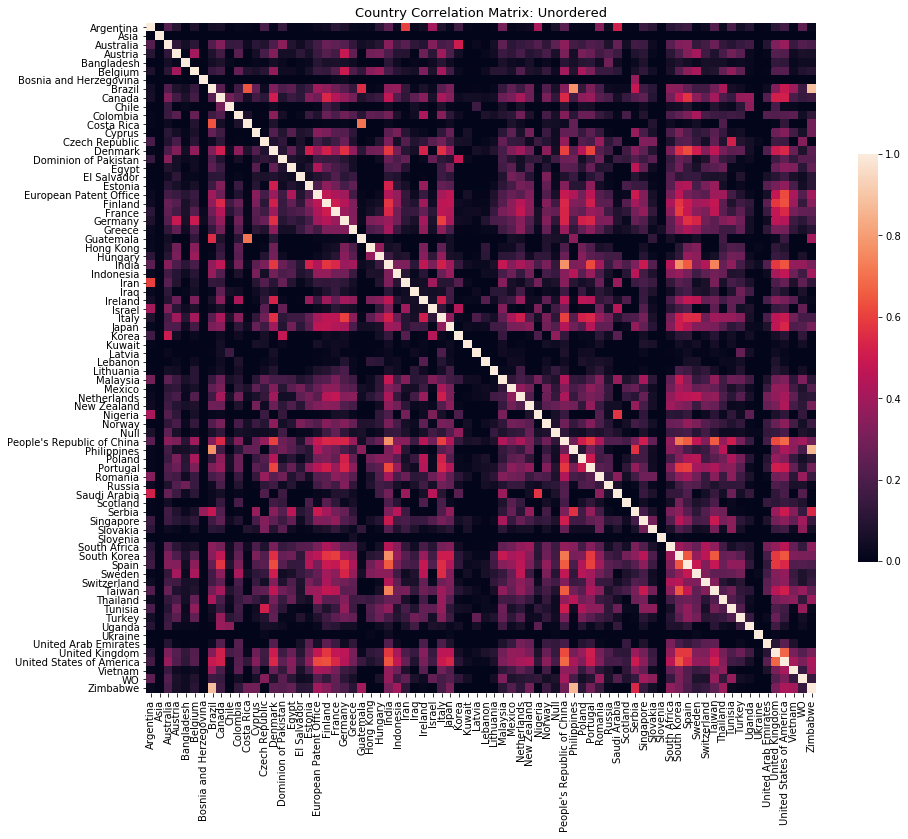A couple of things worth noting in the first visualization:

• All of the values of the heatmap are between 0 and 1.
• A value of 1 indicates the highest level of correlation. For this reason, the diagonal has values equal to 1.(This because it corresponds to the correaltion of a country to itself.)
• The lighter the color of the correlation, the higher the level of correlation.
• If a cell in the heatmap is totally dark, this indicates no correlation at all.

Light Cell - High Correlation: This indicates that the capability profile of the two countries concerned is similar. Which can be interpreted as the countries having "similar" research profiles.

Dark Cell - Low Correlation: This indicates that the capability profile of the two countries concerned is different. Which can be interpreted as the countries having divergent research profiles.

INTERACTIVE PLOTLY VERSION.

### 2.2. Clustered country correlation matrix ¶

In this part, we will create a clustermap of the heatmap produced in the previous sections.

To do this, we use the sns.clustermap function (see documentation here) that produces two things:

• This clustermap also creates a dendogram of the linkages that the algorithm produces.
In :
# plot the clustermap
a = sns.clustermap(country_correlation,  figsize=(15, 15), xticklabels = country_names, yticklabels=country_names)
np.save('Data/correlation_matrix.npy', country_correlation)
np.save('Data/correlation_matrix_names.npy', country_names)
plt.show()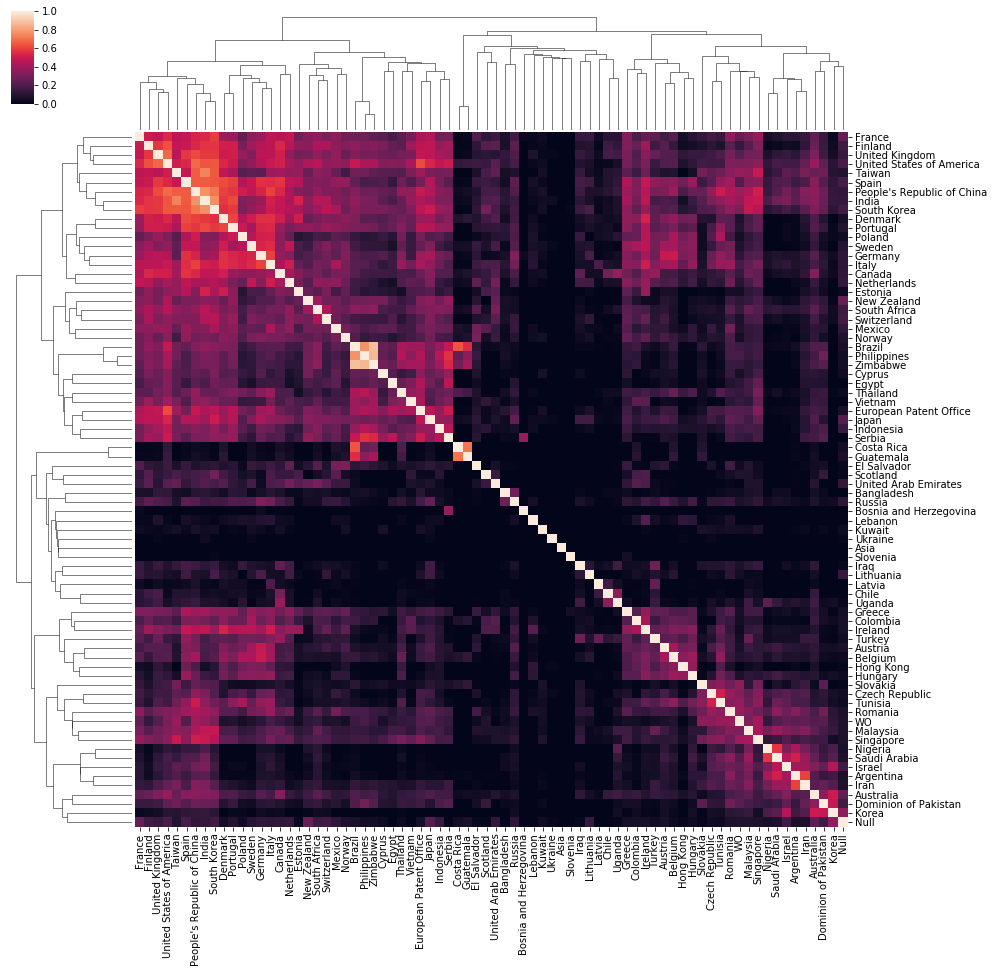Some interesting observations and hypothesis:

• Language matters: UK and USA, for example, are highly correlated. (68% correlation)
• Distance matters: Costa-Rica and Guatemala have a correlation of 71%.
• Other Factors might matter: : Portugal and Denmark for example, have a correlation of 61%.

PS: the percentage of correlation is achieved by multiplying the Pearson correlation index by 100.

### 2.3. Country profiles ¶

Another interesting analysis is understanding how one country relates to other countries itself. This is done by slicing the heatmap produced in the previous section.

By producing a bar plot for every country we can see how it relates to others and possibly find meaningful patterns.

Let's start by selecting a couple of countries:

In :
countries = ['Denmark', 'United Kingdom']


After selecting countries, we can now plot two different profiles.

In :
# for each country selected
for country in countries:

# find the matrix slice
country_index = country_names.index(country)
histogram_data = country_correlation[:, country_index]

# remove the country itself from data and labels
histogram_data = np.delete(histogram_data, country_index)
clean_country_names = np.delete(country_names, country_index)

# sort labels and data
sorted_names = [name for _,name in sorted(zip(histogram_data, clean_country_names))]
histogram_data.sort()

#plot
plt.subplots(1,1,figsize=(15,7))
sns.barplot(np.arange(len(histogram_data)), histogram_data * 100, palette="Reds_d")
plt.xticks(np.arange(len(histogram_data)), sorted_names, rotation=90, fontsize=11)
plt.title('Country Profile: {}'.format(country))
plt.ylabel('Correlation Percentage')
plt.show()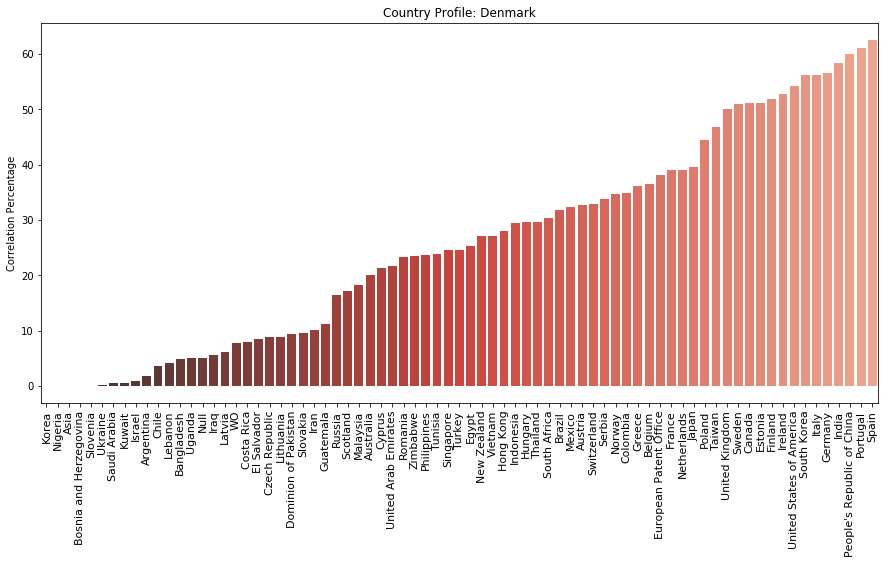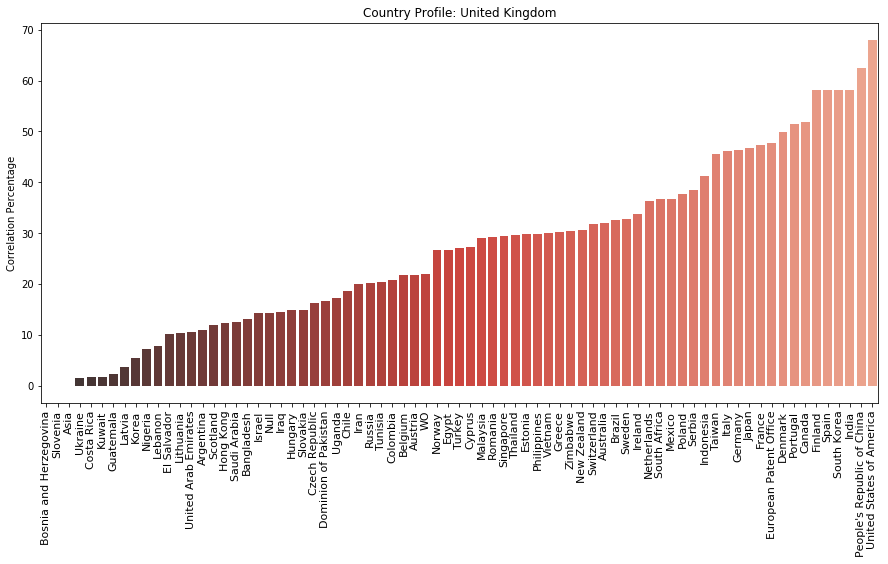INTERACTIVE PLOTLY VERSION

Some important information comes from these profiles:

• Using these profile we can dive deeper into each country to confirm the above hypothesis.
• Looking at UK's profile, the top three countries with highest correlation with it are: USA, China and India. This can mean that language is a very important factor for correlation but also eceonomic development for example.
• Looking at Denmark's profile, the 3 most similar countries are: Spain, Portugal and China. This indicates that there is possibly another important factor at play.

## 3. Contextual Relations ¶

We will now study the relationship between the previously found correlations and other characteristics.

### 3.1. GDP per capita ¶

Let us investigate how the GDP per capita is related to the country capabilities.

The world bank has data available on the GDP per capita for (almost) every country in the world.

Source

Let us investigate what countries or elements are not available in the World Bank database:

In :
data = pd.read_csv('Data/GDP_per_capita.csv', delimiter=';', header=None).as_matrix()
print 'Countries that do not have data:'

for country in country_names:
if country not in data[:, 0]:
print country

Countries that do not have data:
Asia
Null


#### 3.1.1. GDP per capita and capabilities ¶

We delete these entries from the country_correlation matrix (our heatmap) and create an adapted_country_correlation that is equal, without the entries that do not have GDP data available.

In :
countries_not_available = ['Asia', 'Null', 'Korea']
index_countries_not_available = [country_names.index(country) for country in countries_not_available]



Next, we create a matrix where the GDP's are correlated with each other. Following the structure:

• Entry(i,j) is the absolute value of the difference between the GDP per capita of country i and j.
In :
# create the matrix
countries_available = [country for country in country_names if country not in countries_not_available]
countries_available.sort()

# for every entry, calculate the entry
for row in range(len(countries_available)):
country_1 = countries_available[row]
country_1_gdp = float(data[data[:, 0].tolist().index(country_1), 1])
for column in range(len(countries_available)):
country_2 = countries_available[column]
country_2_gdp = float(data[data[:, 0].tolist().index(country_2), 1])

gdps[row, column] = abs(country_1_gdp - country_2_gdp)

gdps_norm = (gdps - np.mean(gdps)) / np.std(gdps)


We can finally plot the GDP per capita difference heatmap.

In :
plt.subplots(1,2,figsize=(17,17))

plt.subplot(121)
sns.heatmap(gdps, square=True, cbar=None, yticklabels=False, xticklabels=False)
plt.title('GDP per capita difference', size=13)

plt.subplot(122)
plt.title('Country capability correlation', size=13)
plt.show()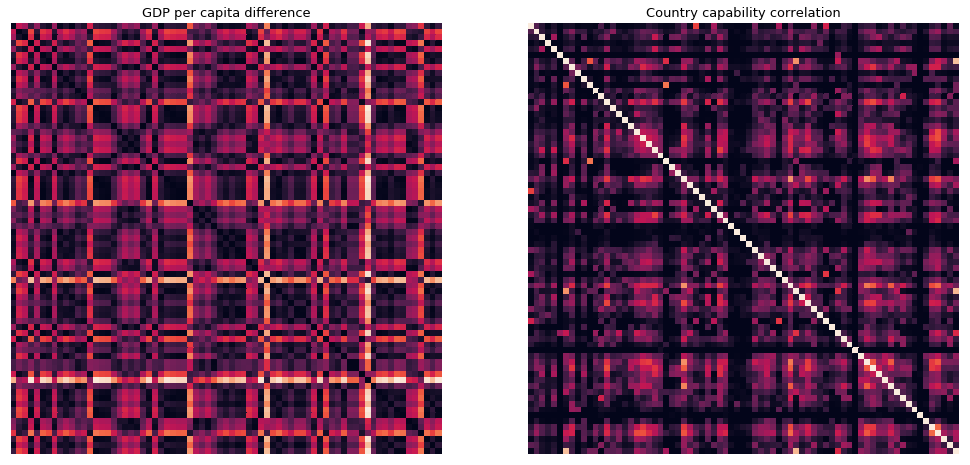Left Figure: The difference in GDP per capita of all of the countries in the database. The lighter the color in the heatmap, the bigger the difference in the GDP per capita between two countries.

Rigth Figure: The knowledge capability correlation between two countries. The lighter the color, the more correlated their capabilities are.

Let's study if these two matrixes are correlated in any way. We start by creating a function that flattens the above matrixes, by takingthe upper triangle and discarting anything below it (including the diagonal).

In :
def custom_flatten(matrix):
rows = matrix.shape
cols = matrix.shape

finalList = []
columnDisplacement = 1
for rowNumber in range(rows):
listToAppend =  matrix[rowNumber, :][columnDisplacement::]
finalList.extend(listToAppend)

columnDisplacement += 1
return finalList


After doing so, we determine two relevant statistics for the correlation study:

In :
rounding = 4
cos_sim = 1 - spatial.distance.cosine(custom_flatten(gdps), custom_flatten(adapted_country_correlation))

print 'Pearson correlation Index: {} (p-value of {})'.format(round(pearson_, rounding), round(p_value, rounding))
print 'Cosine similarity: {}'.format(round(cos_sim, rounding))

Pearson correlation Index: 0.0392 (p-value of 0.0446)
Cosine similarity: 0.5621


The pearson correlation index shows that there is a very small positive correlation between GDP per capita and capability. Moreover, the p-value (< 0.05)comes to show that this assessment is 'statistically relevant'.

#### 3.1.2. Country Profiles and Correlation ¶

Global Correlations - Scatter Plot

As can be noticed above, there are a lot of country pairs that have a 0 capability correlation. This can happen for severall reasons:

• One of the countries does not have a significant number of assets.
• Both countries have a very low number of assets.

To understant the reach of this trait, the same plot is reproduced whyle ignoring the country pairs with 0 capability correlation.

Let us plot some guiding lines that will help us visualize the logic of this plot:

In :
# create global lists for gdps difference and
globalGDP = np.asarray(custom_flatten(gdps))

# eliminate pairs with no correlation
capabilityThreshold = 0.01
globalGDP_notNull = globalGDP[globalCapabilityCorrelation > capabilityThreshold]
globalCapabilityCorrelation_notNull = globalCapabilityCorrelation[globalCapabilityCorrelation  > capabilityThreshold]

print 'A total of {} entries were eliminated from {}.'.format(len(globalCapabilityCorrelation) - len(globalCapabilityCorrelation_notNull), len(globalCapabilityCorrelation))

# scatter plot
fig, ax1 = plt.subplots(figsize=(15,7))
sns.regplot(globalCapabilityCorrelation_notNull, globalGDP_notNull,fit_reg=False, marker=".", color = '#69306d')

x1 = 0.0
x2 = 0.1
y1 = 10000
y2 = 0

for k in range(9):
plt.plot([x1, x2], [y1, y2], ls="--", c='grey', lw=0.8)
y1 = y1 + 10000
x2 = x2 + 0.1

plt.xlabel('Capability Correlation of country pairs')
plt.ylabel('GDP per capita difference of country pairs')
plt.title('Capability Correlation Vs. GDP per capita difference: Global (Excluded if capability less than {})'.format(capabilityThreshold))
plt.show()

A total of 633 entries were eliminated from 2628.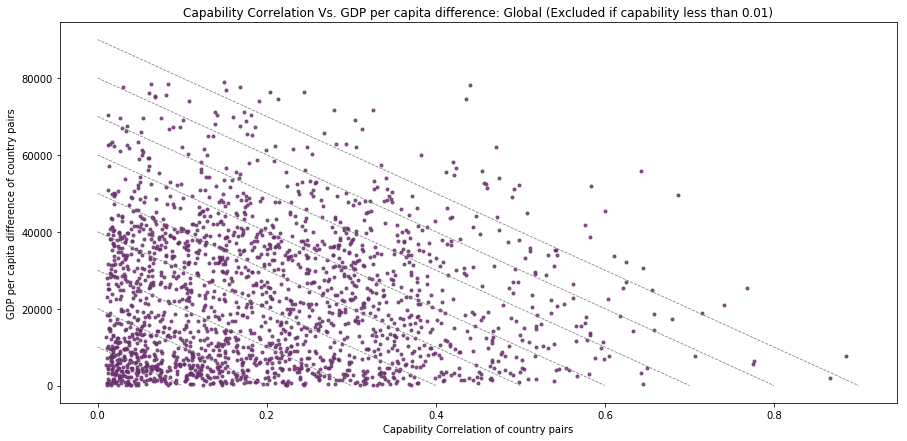Let us investigate some outliers:

In :
gdpPerCapitaLowerLimit = 45000
capabilityCorrelationLowerLimit = 0.5

print 'Outliers'
for i in range(len(countries_available)):
for j in range(len(countries_available)):
if np.triu(gdps, 1)[i,j]>gdpPerCapitaLowerLimit and np.triu(adapted_country_correlation, 1)[i,j]> capabilityCorrelationLowerLimit:
print countries_available[i],'-', countries_available[j]


Outliers
Denmark - India
Denmark - People's Republic of China
India - United States of America
People's Republic of China - United States of America


Global Correlations- Density Plot

In :
# density plot
fig, ax1 = plt.subplots(figsize=(15,7))
sns.kdeplot(globalCapabilityCorrelation, globalGDP, cmap="Blues", shade=True, cbar = True)
plt.xlabel('Capability Correlation of country pairs')
plt.ylabel('GDP per capita difference of country pairs')
plt.title('Capability Correlation Vs. GDP per capita difference density plot: Global')
plt.show()

plt.subplots(1,2,figsize=(15,7))

plt.subplot(121)
plt.title('Global correlation distribution')

plt.subplot(122)
plt.title('Global GDP per capita difference distribution')
plt.show()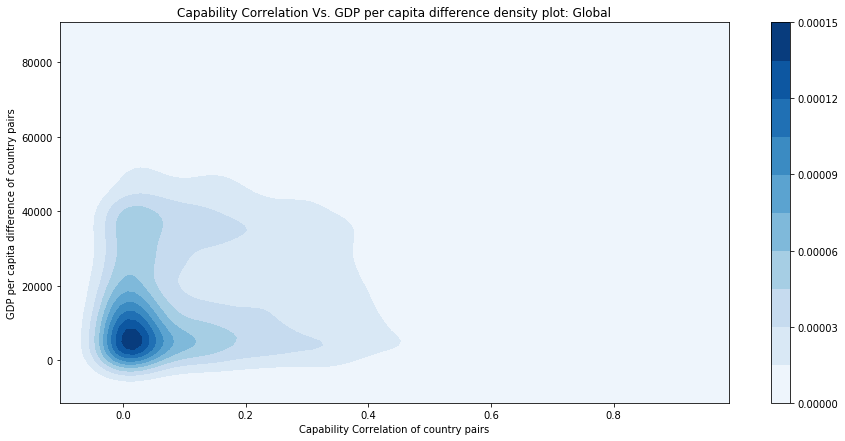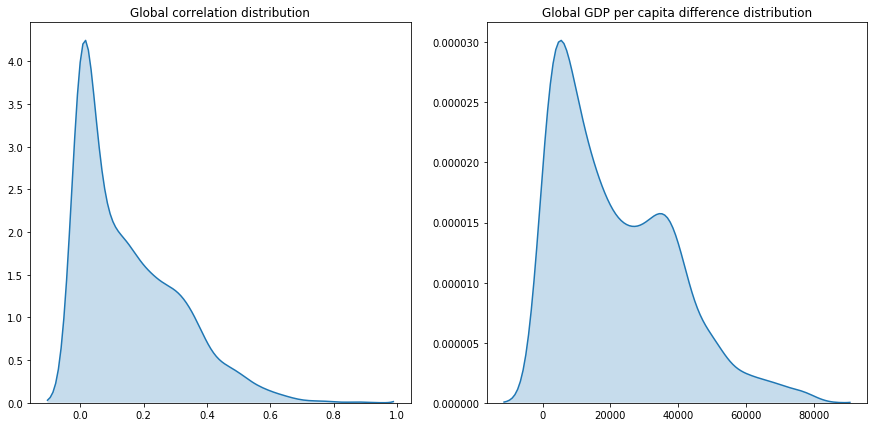#### 3.1.3. Using the average GDP per capita ¶

In this part of the analysis we will introduce the average gdp per capita between two countries, let's start by creating the matrix.

In :
# create the matrix
countries_available = [country for country in country_names if country not in countries_not_available]
countries_available.sort()

# for every entry, calculate the entry
for row in range(len(countries_available)):
country_1 = countries_available[row]
country_1_gdp = float(data[data[:, 0].tolist().index(country_1), 1])
for column in range(len(countries_available)):
country_2 = countries_available[column]
country_2_gdp = float(data[data[:, 0].tolist().index(country_2), 1])

gdps_average[row, column] = (country_1_gdp + country_2_gdp) / 2.0

In :
plt.subplots(1,1,figsize=(13, 13))
plt.subplot(111)
sns.heatmap(gdps_average, square=True, cbar=True, yticklabels=countries_available, xticklabels=countries_available)
plt.title('GDP per capita average', size=13)
plt.show()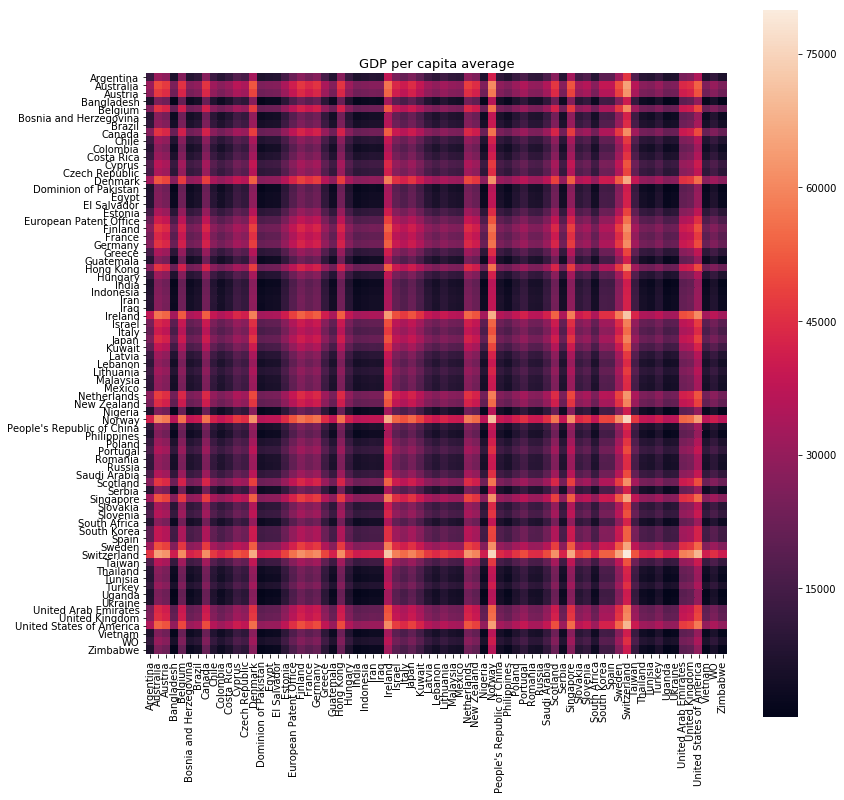In :
globalGDP = np.asarray(custom_flatten(gdps))
globalGdpAverage = np.asarray(custom_flatten(gdps_average)) / 800

# eliminate pairs with no correlation
capabilityThreshold = 0.01
globalGDP_notNull = globalGDP[globalCapabilityCorrelation > capabilityThreshold]
globalCapabilityCorrelation_notNull = globalCapabilityCorrelation[globalCapabilityCorrelation  > capabilityThreshold]
globalGdpAverage_notNull = globalGdpAverage[globalCapabilityCorrelation  > capabilityThreshold]

# scatter plot
fig, ax1 = plt.subplots(figsize=(15,7))
plt.scatter(globalCapabilityCorrelation_notNull, globalGDP_notNull, s=globalGdpAverage_notNull, marker="o")
plt.xlabel('Capability Correlation of country pairs')
plt.ylabel('GDP per capita difference of country pairs')
plt.title('Capability Correlation Vs. GDP per capita difference: Global (Excluded if capability less than {})'.format(capabilityThreshold))
plt.show()